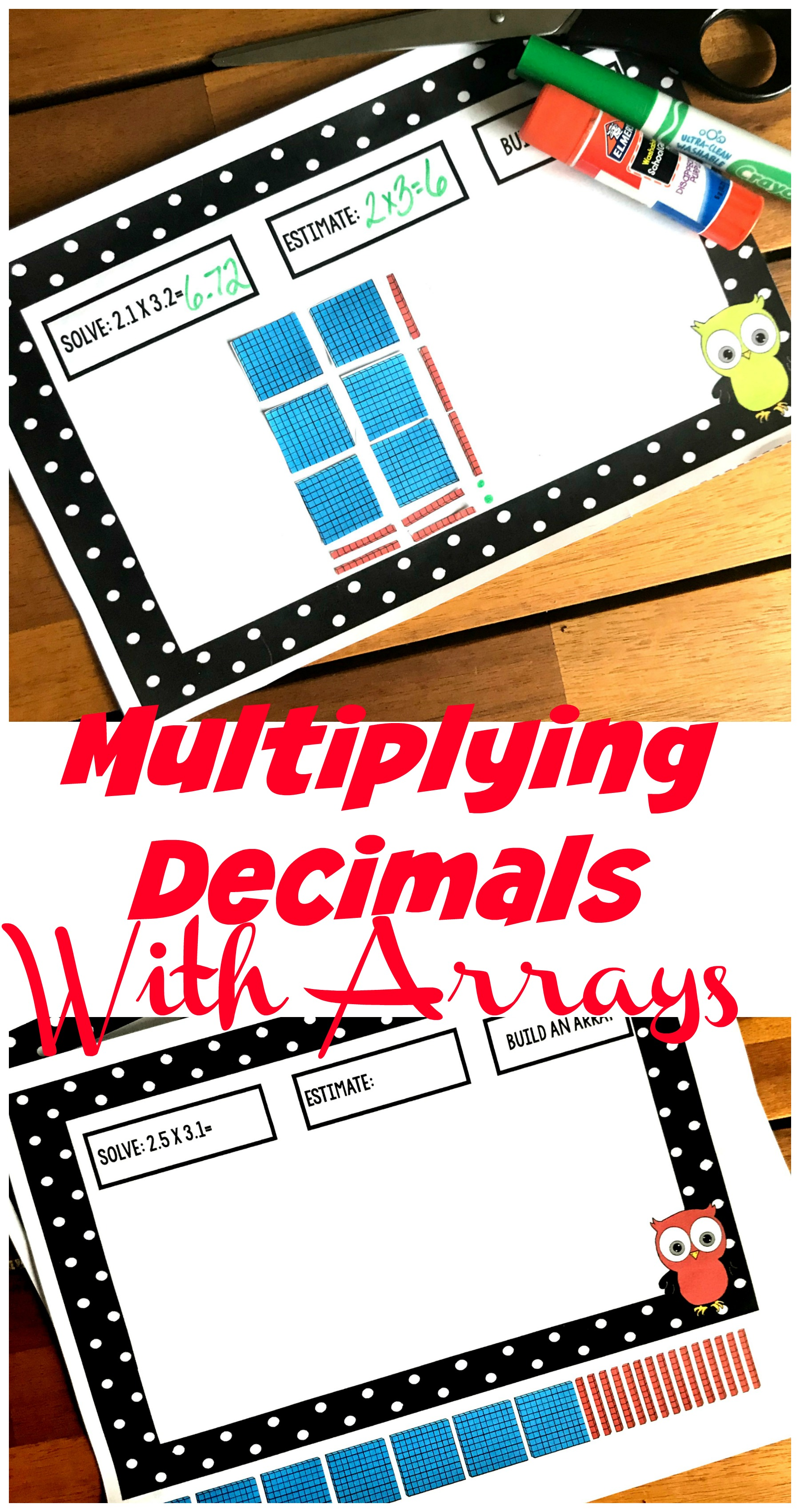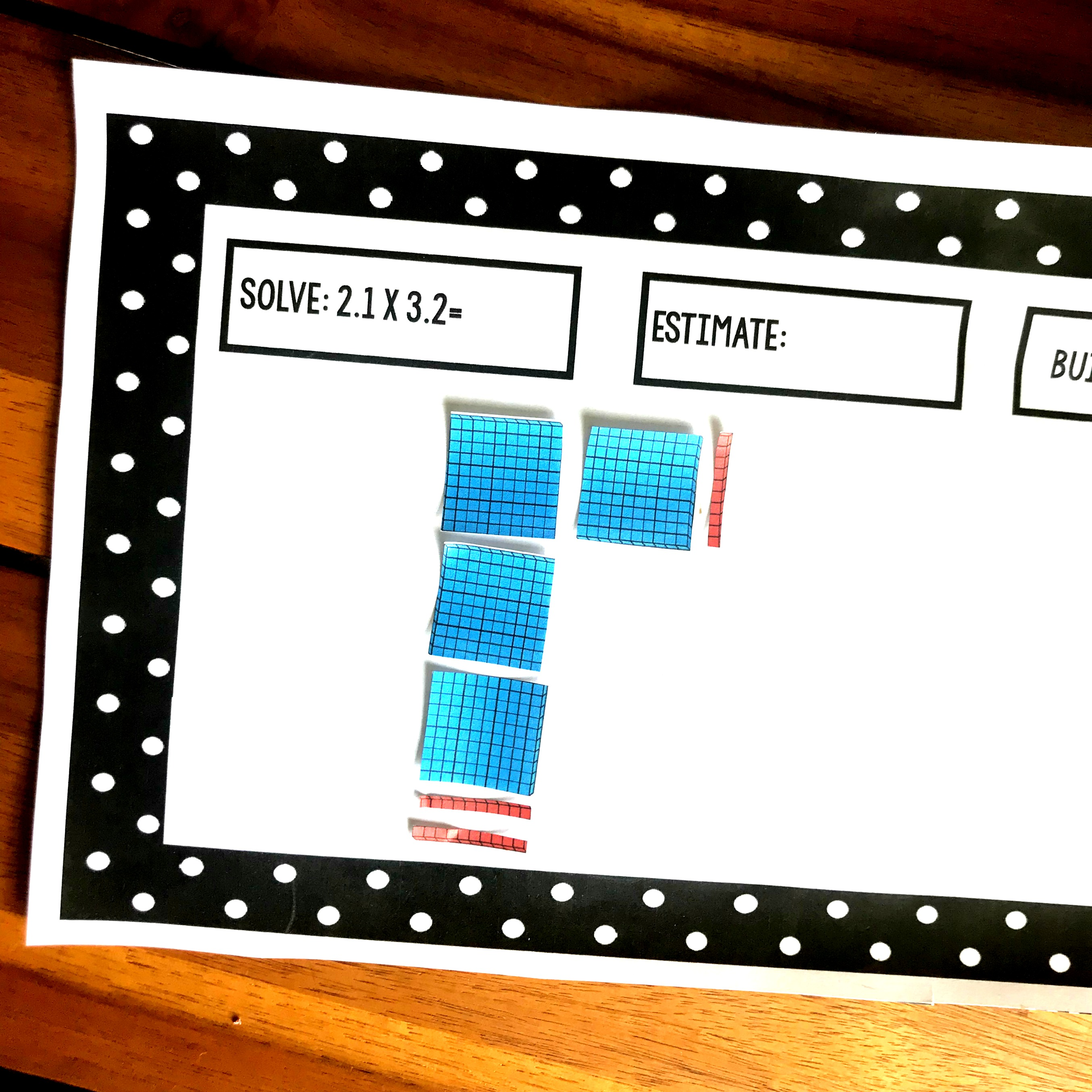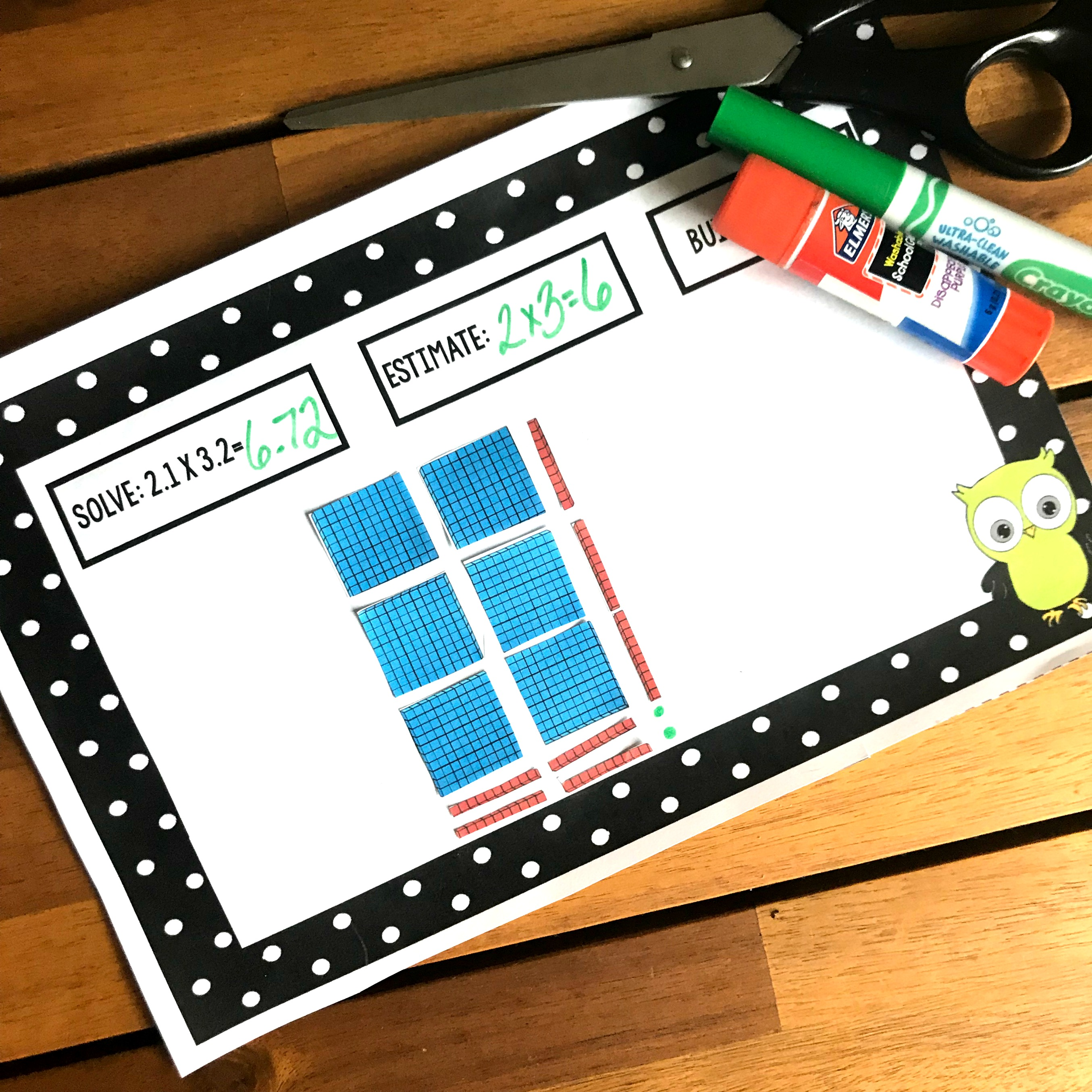Home » Math » How to Model Multiplying Decimals With Arrays (Free Printable)

How to Model Multiplying Decimals With Arrays (Free Printable)

This fun interactive worksheet has children learning how to model multiplying decimals with arrays. It’s a great way for children to visualize what is happening when they multiply decimals.I have very strong feelings on how math should be taught. And if you have read much of my blog, you know what they are.

I believe math that math shouldn’t be filled with worksheets for practice, but fun games. But before those games are pulled out, the math should be taught in a visual way. Our kiddos should be playing with manipulatives, drawing arrays, working on number lines, and so much more.

It is not that we want our children to ALWAYS solve the problems that way. But allowing them to play and manipulate objects to solve a problem builds a number sense that will help them correctly solve problems when they are just doing the formula.

And that is what today is all about. It allows children to learn how to model multiplying decimals with arrays. It is a great place to start when teaching this concept.

Prep-Work

Ok, you will love this! There isn’t any prep work!!!!

1. Print of the pages you want to use.
2. Then, provide glue, scissors, a marker, and a pencil.

That’s it! You are ready to go!!!

Model Multiplying Decimals

Alright, let’s work through one of these task cards.First, we want our children to estimate.

Our multiplication problem is 2.1 x 3.2.

2.1 rounds down to 2 and 3.2 rounds down to 3. Soooooo, our estimate is 6. 2 x 3 = 6.

Next, we focus on modeling decimal multiplication.

We will begin by modeling 2.1.  The flats equal one whole, and the long equal one-tenth. Therefore, I need two flats and one long.Now, we model 3.2. And instead of doing it horizontally, we will do it vertically. Now, I already have one whole on the page, and I will use this flat to represent one of my three wholes. Soooo, I only need to add two more wholes.

Now that the two wholes are added, I finish up modeling 3.2, by adding 2 longs.Finally, I continue adding flats and longs to make a rectangle. I will use a marker to represent any cubes that need to be added.Solving A Multiplying Decimals Problem

Now that our beautiful array is done, it is time to solve. And we do this by counting up the base ten blocks we used.

Let’s start with the flats. Remember each flat equals one whole. Since we used 6, we have six ones.

Now we move onto the longs. These represent the number in the tenths place. There are seven of them so I know that I have seven-tenths.

Lastly, we move to the cubes. They represent the number in the thousandths place. Four dots were placed to finish off the rectangle, so I have four hundredths.

Altogether, I have 6.72

3.2 x 2.1 = 6.72This is a wonderful way to work through a decimal problem, and I hope it helps your children who learn visually.

You’ve Got This,

Rachel

You may also like:

Multiplying Decimal PuzzlesFive multiplying decimal activitiesThis is a subscriber freebie. If you are already a subscriber, check the most recent email for the latest password. If you aren’t a subscriber, sign up below and the password will be provided in the first email you receive. You will also get \$2 off to use the You’ve Got This Store.

You’ll find it under the decimal section!